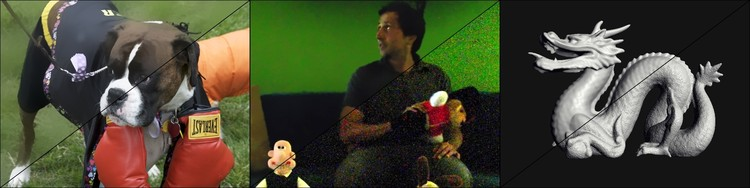Gaussian KD-Trees for Fast High-Dimensional Filtering

 Andrew Adams Natasha Gelfand Jennifer Dolson Marc Levoy

SIGGRAPH 2009We use a Monte-Carlo sampled kd-tree we call the Gaussian kd-tree for various filtering tasks. Our method applies to bilateral filtering of image (left), non-local means of bursts of images (middle), and denoising geometry (right).

Abstract

We propose a method for accelerating a broad class of non-linear filters that includes the bilateral, non-local means, and other related filters. These filters can all be expressed in a similar way: First, assign each value to be filtered a position in some vector space. Then, replace every value with a weighted linear combination of all values, with weights determined by a Gaussian function of distance between the positions. If the values are pixel colors and the positions are (x, y) coordinates, this describes a Gaussian blur. If the positions are instead (x, y, r, g, b) coordinates in a five-dimensional space-color volume, this describes a bilateral filter. If we instead set the positions to local patches of color around the associated pixel, this describes non-local means. We describe a Monte-Carlo kd-tree sampling algorithm that efficiently computes any filter that can be expressed in this way, along with a GPU implementation of this technique. We use this algorithm to implement an accelerated bilateral filter that respects full 3D color distance; accelerated non-local means on single images, volumes, and unaligned bursts of images for denoising; and a fast adaptation of non-local means to geometry. If we have n values to filter, and each is assigned a position in a d-dimensional space, then our space complexity is O(dn) and our time complexity is O(dn log n), whereas existing methods are typically either exponential in d or quadratic in n.

Paper

Video

Supplemental Material

Implementations

Here is the GPU implementation of the paper, which runs on linux with NVIDIA's cuda installed. There's a readme.txt inside the package to get you started: Linux Binary and Source

Here is the CPU implementation of the paper, including example code for computing bilateral filters: Cygwin Binary and Cross-Platform Source.

The CPU implementation is also included in ImageStack, including operations that use it for bilateral filtering, joint bilateral filtering, and non-local means.